## Locating and Ordering Fractions and Mixed Numbers on the Number Line

### Learning Outcomes

• Locate fractions and mixed numbers on the number line
• Order fractions and mixed numbers using lists and inequalities

Now we are ready to plot fractions on the number line. This will help us visualize fractions and understand their values.

Let us locate ${\dfrac{1}{5}, \dfrac{4}{5}}, 3, 3{\dfrac{1}{3}, \dfrac{7}{4}, \dfrac{9}{2}}, 5$, and ${\dfrac{8}{3}}$ on the number line.

We will start with the whole numbers $3$ and $5$ because they are the easiest to plot.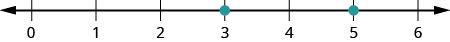The proper fractions listed are ${\dfrac{1}{5}}$ and ${\dfrac{4}{5}}$. We know proper fractions have values less than one, so ${\dfrac{1}{5}}$ and ${\dfrac{4}{5}}$ are located between the whole numbers $0$ and $1$. The denominators are both $5$, so we need to divide the segment of the number line between $0$ and $1$ into five equal parts. We can do this by drawing four equally spaced marks on the number line, which we can then label as ${\dfrac{1}{5},\dfrac{2}{5},\dfrac{3}{5}}$, and ${\dfrac{4}{5}}$.

Now plot points at ${\dfrac{1}{5}}$ and ${\dfrac{4}{5}}$.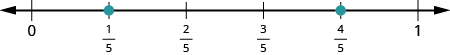The only mixed number to plot is $3{\dfrac{1}{3}}$. Between what two whole numbers is $3{\dfrac{1}{3}}$? Remember that a mixed number is a whole number plus a proper fraction, so $3{\dfrac{1}{3}}>3$. Since it is greater than $3$, but not a whole unit greater, $3{\dfrac{1}{3}}$ is between $3$ and $4$. We need to divide the portion of the number line between $3$ and $4$ into three equal pieces (thirds) and plot $3{\dfrac{1}{3}}$ at the first mark.Finally, look at the improper fractions ${\dfrac{7}{4},\dfrac{9}{2}}$, and ${\dfrac{8}{3}}$. Locating these points will be easier if you change each of them to a mixed number.

${\dfrac{7}{4}}=1{\dfrac{3}{4}},{\dfrac{9}{2}}=4{\dfrac{1}{2}},{\dfrac{8}{3}}=2{\dfrac{2}{3}}$

Here is the number line with all the points plotted.### Example

Locate and label the following on a number line: ${\dfrac{3}{4},\dfrac{4}{3},\dfrac{5}{3}},4{\dfrac{1}{5}}$, and ${\dfrac{7}{2}}$.

Solution:
Start by locating the proper fraction ${\dfrac{3}{4}}$. It is between $0$ and $1$. To do this, divide the distance between $0$ and $1$ into four equal parts. Then plot ${\dfrac{3}{4}}$.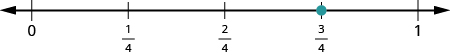Next, locate the mixed number $4{\dfrac{1}{5}}$. It is between $4$ and $5$ on the number line. Divide the number line between $4$ and $5$ into five equal parts, and then plot $4{\dfrac{1}{5}}$ one-fifth of the way between $4$ and $5$ .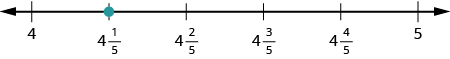Now locate the improper fractions ${\dfrac{4}{3}}$ and ${\dfrac{5}{3}}$. It is easier to plot them if we convert them to mixed numbers first.

${\dfrac{4}{3}}=1{\dfrac{1}{3}},{\dfrac{5}{3}}=1{\dfrac{2}{3}}$
Divide the distance between $1$ and $2$ into thirds.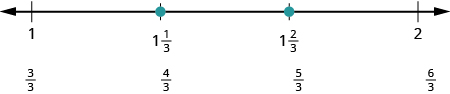Next let us plot ${\dfrac{7}{2}}$. We write it as a mixed number, ${\dfrac{7}{2}}=3{\dfrac{1}{2}}$ . Plot it between $3$ and $4$.The number line shows all the numbers located on the number line.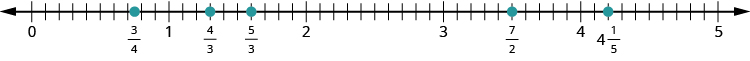### try it

Watch the following video to see more examples of how to locate fractions on a number line.

We previously defined the opposite of a number. It is the number that is the same distance from zero on the number line but on the opposite side of zero. We saw, for example, that the opposite of $7$ is $-7$ and the opposite of $-7$ is $7$.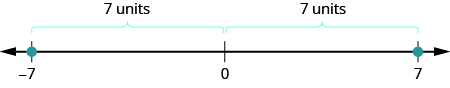Fractions have opposites, too. The opposite of ${\dfrac{3}{4}}$ is $-{\dfrac{3}{4}}$. It is the same distance from $0$ on the number line, but on the opposite side of $0$.Thinking of negative fractions as the opposite of positive fractions will help us locate them on the number line. To locate $-{\dfrac{15}{8}}$ on the number line, first think of where ${\dfrac{15}{8}}$ is located. It is an improper fraction, so we first convert it to the mixed number $1{\dfrac{7}{8}}$ and see that it will be between $1$ and $2$ on the number line. So its opposite, $-{\dfrac{15}{8}}$, will be between $-1$ and $-2$ on the number line.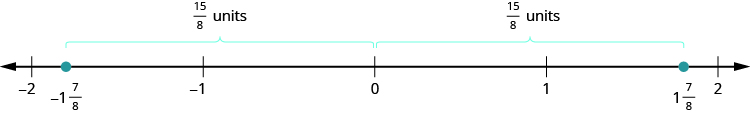### Example

Locate and label the following on the number line: ${\dfrac{1}{4}},-{\dfrac{1}{4}},1{\dfrac{1}{3}},-1{\dfrac{1}{3}},{\dfrac{5}{2}}$, and $-{\dfrac{5}{2}}$.

### Try it

In the next video we give more examples of how to locate negative and positive fractions on a number line.

## Order Fractions and Mixed Numbers

We can use the inequality symbols to order fractions. Remember that $a>b$ means that $a$ is to the right of $b$ on the number line. As we move from left to right on a number line, the values increase.

### Example

Order each of the following pairs of numbers, using $<$; or $>:$

1. $-{\dfrac{2}{3}}$ ____ $- 1$
2. $-3{\dfrac{1}{2}}$ ____ $- 3$
3. $-{\dfrac{3}{7}}$ ____ ${\dfrac{3}{8}}$
4. $-2$ ____ ${\dfrac{-16}{9}}$

### Try it

In the following video we show another example of how to order integers, fractions and mixed numbers using inequality symbols.

## Contribute!

Did you have an idea for improving this content? We’d love your input.